# 射频 - 谐振电路 - 负载Q值 🚧

• 源阻抗 $$R_s$$
• 负载电阻 $$R_L$$
• 上一章提到的元器件的 Q 值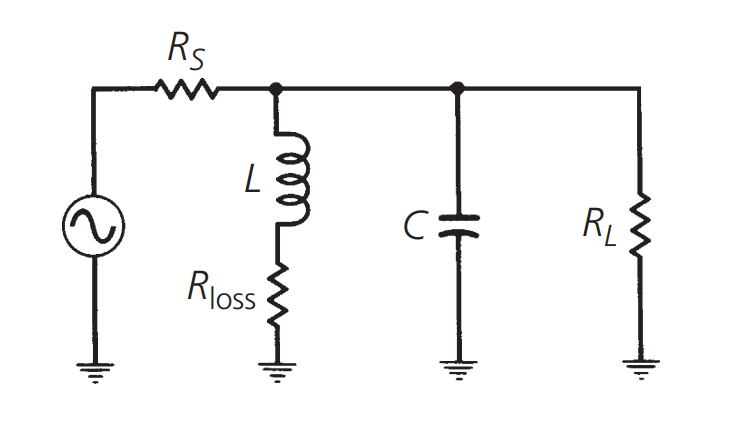## $$R_s$$ 和 $$R_L$$ 对负载 Q 的影响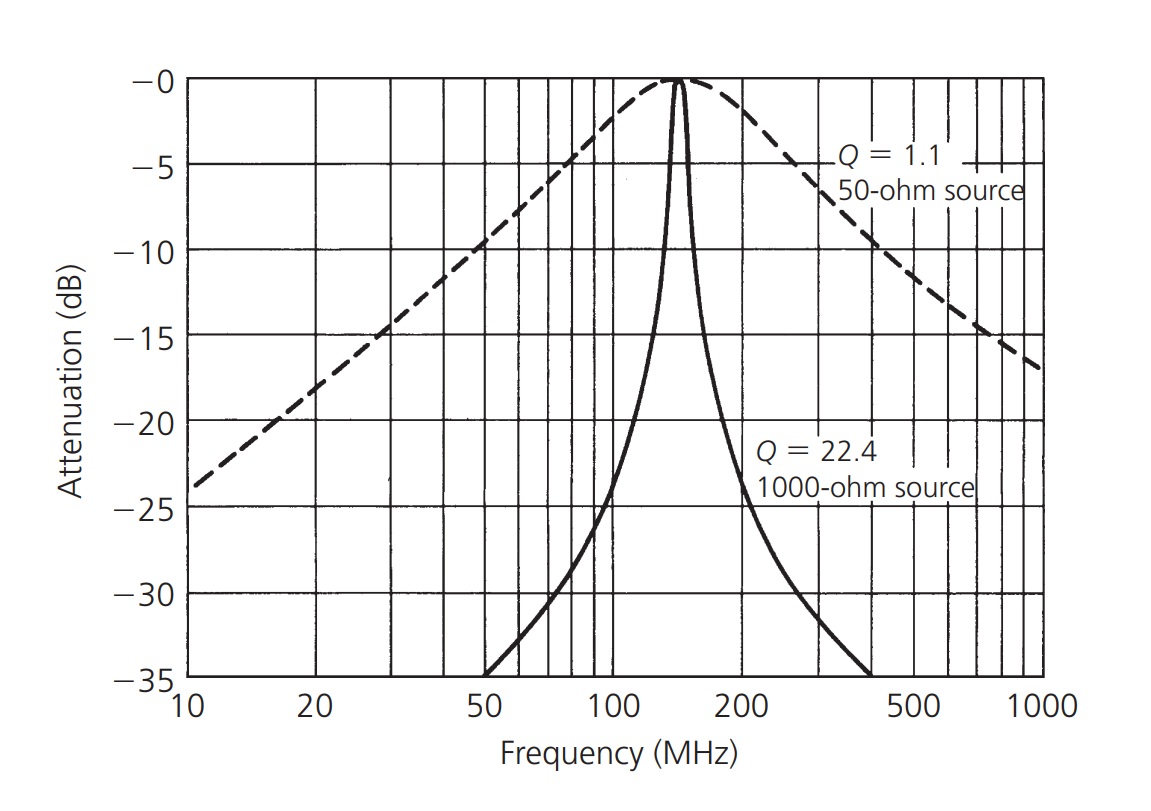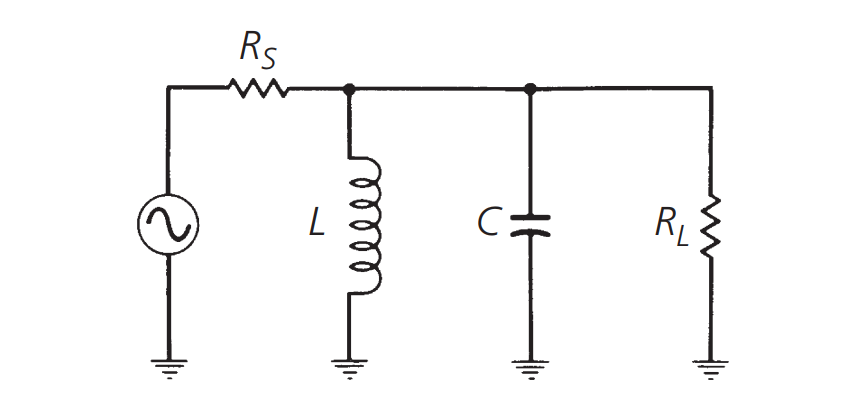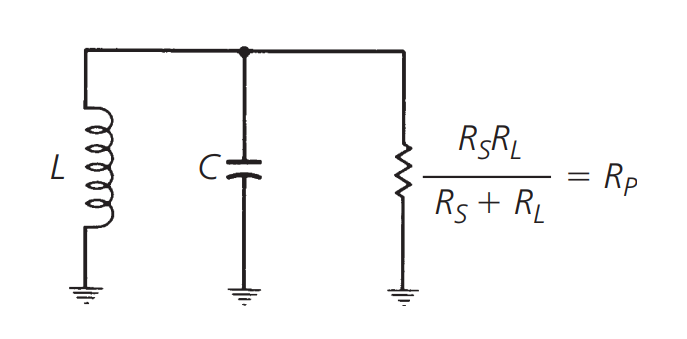$Q=\frac{R_p}{X_p}$

e.g. 如果我们要设计一个谐振电路，使其在 150Ω 的源阻抗和 1000Ω 的负载阻抗条件下运行。在 50 MHz 的谐振频率下，负载 Q 须等于 20。假设无损耗元件且无阻抗匹配。那么我们可以得出 $$R_p=130Ω$$，根据上文公式，$$X_p=\frac{R_p}{Q}=\frac{130}{20} =6.5Ω$$，又因为 $$X_p=\omega L=\frac{1}{\omega C}$$，因此，可选 20.7nH 的电感、489.7pF 的电容。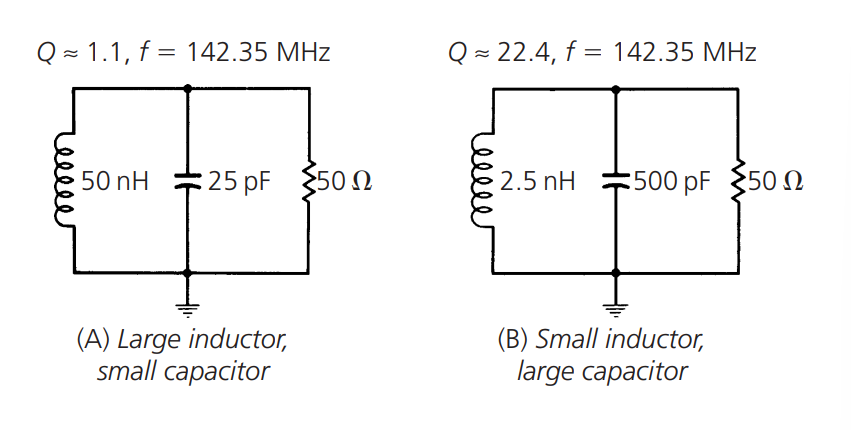1. 选择源阻抗和负载阻抗的最佳值。
2. 选择优化 Q 的 L 和 C 的分量值。

## 元器件的 Q 值对负载 Q 值的影响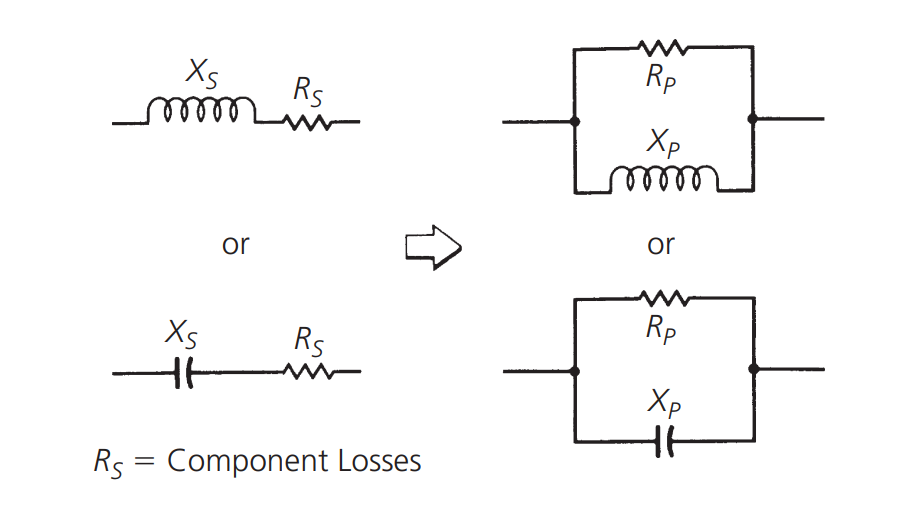## 参考与致谢

• 《RF-Circuit-Design(second-edition)_Chris-Bowick》